RUSSIAN JOURNAL OF EARTH SCIENCES, VOL. 12, ES5001, doi:10.2205/2012ES000520, 2012

# Classification of solar X-ray flares on X-ray measurements on board geostationary satellites "Elektro"

A. A. Nusinov, V. V. Katyushina

Institute of Applied Geophysics, Moscow, Russia

.

### Abstract

Fluxes of soft $X$-ray radiation (XR) are the most important indicator of space weather. Currently, the standard is to classify the power of solar flares on the basis of XR measurements in 0.1–0.8 nm waverange onboard geostationary satellites GOES. As a part of the monitoring of conditions in the near-Earth space on Russian satellite of series "Elektro" a Geiger counter with an aluminum filter was used as the XR detector, the bandwidth of the detector is 0.05–0.4 nm. We propose a method of classification of solar flares and the calculation of the spectrum for the simulation of the ionosphere based on the satellite "Elektro" measurements. The method is based on the algorithm for calculating the flow of XR in the 0.1–0.8 nm based on the "Elektro" measurements data, and then use the model spectra of $X$-ray emission of the flare.

### Introduction

Results of soft $X$-ray radiation fluxes (SXR) measured on board of satellites are widely used in scientific and applied researches and are one of the most important indicators of space weather. Systematic monitoring of soft $X$-ray radiation has proceeded successfully for more than forty years with the help of the equipment, installed on the board the American Geostationary Operational Environmental Satellite (GOES) by US National Oceanic and Atmospheric Administration (NOAA). The applied $X$-ray detectors for these purposes are ionization chambers, which make it possible to measure the intensity of the radiation in the two wide integral channels $1 - 8$ Å (1.5–12.4 keV) and 0.5–4.0 Å (3–25 keV).

Soft $X$-ray radiation measurements still have not been carried out in Russia. Within the scope of the creation of space segment of environment monitoring in the near space, it is supposed to create systems of the Sun soft $X$-ray radiation patrol observations. In particular, the installation is planned on the solar battery panels of the spacecraft "Elektro-L" No. 1, 2, 3 with the purpose of radiation monitoring. In what follows it is also planned to install $X$-ray detectors on the spacecraft "Elektro-M". A Geiger counter with an aluminum filter is applied in the instrument as a detector.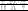Table 1

The system of estimated power of solar fluxes according to $X$-ray radiation is admitted all over the world and nowadays is based on radiation flux changes in the range $0.1 - 0.8$ nm (Table 1), see, e.g. [Altyntsev et al., 1982].

The frequency of major flares (more than $2 \times 10^{-3}$ erg cm$^{-2}$ s$^{-1}$), which causes sudden ionospheric disturbances (SID), is a linear function of sunspot area and a velocity of change area. An importance of a flare depends on flare area in the group and geometry of the group: the more it is complicated the more probable is flare appearance, caused SID.

During solar flares $X$-ray flux dramatically increased and, consequently, the rate of ionization of the upper atmosphere, which leads to significant changes in the ionosphere (see, e.g. [Nusinov and Chulankin, 1997]). For calculations of changes in the state of the ionosphere during solar flares (especially the absorption of radio waves, and the full content of the electrons in a single column) the spectrum of soft $X$-rays is required. For this kind of aerodynamic calculations in [Qian et al., 2011] the aerodynamic spectrum of $X$-ray flares was obtained, in which soft $X$-ray energy flux SXR $I_{0.1-0.8}$ in the wavelength range 0.1–0.8 nm was the input parameter

\begin{eqnarray} I_{0-\lambda} = C (\lambda) I_{0.1-0.8}^{D(\lambda)} \end{eqnarray}

where

\begin{eqnarray} C(\lambda) = C_0 \exp (-C_1/ \lambda^{C_2}) \end{eqnarray} \begin{eqnarray} D(\lambda) = D_0 + D_1/ \lambda \end{eqnarray}

$\lambda$ in nanometer, $I_{\lambda}$ in mW m$^{-2}$, and in (1)–(3) ratios are defined as follows: $C_0 = 73.8$, $C_1 = 3.80$, $C_2= 0.36$, $D_0 = 0.848$, $D_1 = 0.167$. The energy flux in any spectral interval from $\lambda_1$ to $\lambda_2$ can be calculated according to (3) how, where $I_\lambda$ – the total flux of radiation at wavelengths shorter than $\lambda$.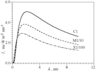Figure 1

For example, Figure 1 shows the differential energy spectra for the flares of classes C1, M1 and X1, calculated by the equations (1)–(3). For the convenience of fluxes divided by 10 for M1 flares and 100 – class X1.

### The Relationship Between X-ray Flux During Flares

Here we consider the relation between the $I_{0.1 - 0.8}$ and $I_{0.05 - 0.3}$ during solar flares. In both cases the analysis was performed on the material of long-term flux measurements onboard the satellite series GOES USA [Kreplin et al., 1977]. Note that in [Chulankin, 1997] by the results of the measurements onboard the SOLRAD satellite during flares, the relation was $I_{0.05 - 0.3} = 2.67 \times I_{0.1 - 0.8}^{1.41}$ mW m$^{-2}$. The results of earlier studies [Donnelly, 1976] according to the SMS-1 satellite, gave a slightly different result: $I_{0.05 - 0.3} = 5.6 \times I_{0.1 - 0.8}^{1.4}$. It should be noted that the notation $I_{0.05 - 0.3}$ and $I_{0.05 - 0.4}$ given by different authors are the same, and differences in the writing reflect only the views of authors about the differences between the measured wavelengths.

In processing of the results of GOES satellite measurements data are used with a 5-minute averaging. Only impulsive flares were selected. Monthly periods were selected for the analysis. When there were at least a few $X$-ray flares of class X (the order of $10^{-4}$ W m$^{-2}$ in the band $0.1 -0.8$ nm) $X$-ray flares of classes X and M were recorded (the order of $10^{-5}$ W m$^{-2}$ in the band $0.1 -0.8$ nm). For periods when there were no flares of class X, outbreaks of class M and C were recorded. (About $10^{-6}$ W m$^{-2}$ in the band $0.1 - 0.8$ nm). For several periods of very low activity, when there were no flares of class M, small flares of class B were recorded (about $10^{-7}$ W m$^{-2}$ in the band $0.1 - 0.8$ nm). Flares with simultaneous maximum values, and flares when the "short wave" band reached a maximum value somewhat (within five minutes) before the "long wave" were not divided. Both bands were selected with maximum value. The results of measurements in 1992, 1996, 1999, 2000, 2001, 2002, 2003 are considered.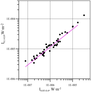Figure 2

Figure 2 shows an example of flow depending on the "long wave" band of the flux in the "short wave" band for February 1992. Along the coordinate axes on a logarithmic scale flow units are shown above W m$^{-2}$. It has seen that the desired relationship is well approximated by a power function:

\begin{eqnarray} I_{0.1-0.8} = A \times I_{0.05-0.4}^B \end{eqnarray}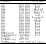Table 2

Table 2 presents the coefficients $A$ and $B$ for all the considered periods. In the first column – the period of measurement, in the second and third ones – the coefficients $A$ and $B$, in the fourth – $X$-class flares are recorded in the periods indicated.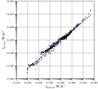Figure 3

In the last row of Table 2 the coefficients, obtained from the generalized data of the above measurements are given. Figure 3 shows this dependence. The results of earlier measurements processing onboard the satellite SMS and SOLRAD [Chulankin, 1997; Donnelly, 1976] are also presented. Table 2 allows us to conclude that there is a considerable (up to an order of a magnitude) scatter of values of the coefficients that characterize the relative magnitude of flows (factor A). This can be due to the variability of relative calibration in these bands on the various devices of GOES series. However, differences in factors B that determine the slope of the line approximating the dependence are small. The same conclusion can come and while considering Figure 3. It shows the dependence of the flow in the "long wave" ($0.1 - 0.8$ nm) band on the flux in the "short wave" band ($0.05 - 0.4$ nm) for all periods presented in Table 1, grouped into a single array. All points from the lowest values, which characterize from virtually calm conditions to super flares (of $10^{-3}$ W m$^{-2}$ s$^{-1}$).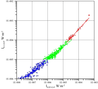Figure 4

However, on a closer inspection you can see the tendency to decrease in the ratio with decreasing energy of the flare. To test this idea with all the data in Table 1, flare classes C, M and X were selected individually. In Figure 4 they are represented by blue, green and red colors. Obtained coefficients $A$ and $B$ are given in Table 3.

It is evident that there is the trend that was mentioned earlier. However, now it is difficult to determine whether it is true or is it the effect of more precise calibration of instruments in recent years, as earlier, such large flares, as in the maximum of 23 cycles, were not observed, and in 1859, when the biggest solar flare was observed, it was still very far from the era of satellites.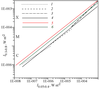Figure 5

Figure 5 allows us to estimate the maximum error of the model, constructed using these data. It gives a diagram with two sets of factors: the maximum and minimum values. It also presents the results of previous studies [Chulankin, 1997; Donnelly, 1976]. It is evident that for the flares of class X the error can reach 100% and P differences are relatively small $(\sim 10-15$%).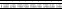Table 4

Comparing the 4, 5 and 6 in Figure 5, we can conclude that at different periods of calibration of measuring instruments onboard the satellites series of SMS, SOLRAD and GOES differed markedly, that leads to differences of up to 100% between the calculated values of the flow of $I_{0.1-0.8}$, derived on the basis of current measurements and calculations on the basis of 1970–1980's. Starting from (4) and the coefficients in Table 2, we can determine the $X$-ray class of flares using planned "Electro-L" spacecraft measurements of the radiation flux in flares $0.05-0.4$ nm. Data for the estimates are presented in Table 4.

Each row of Table 4 corresponds to the class flare due to the classification of NOAA (C, M or X), and the column is its numerical characteristics. Data in the table give us a possibility to compare planned measurements of CMB with the simultaneous GOES measurements.

### Conclusions

1. As a result of the analysis of Solar $X$-ray data flares for the last two decades it was obtained the relation which allows in a general way to calculate the spectral function of solar radiation during flares of an arbitrary class. The calculation of the spectrum requires knowledge of the source parameters – flare $X$-ray flux in the range of 0.05–0.4 nm.

2. The obtained results allow comparing future measurements of soft $X$-radiation on board the satellites of space segment monitoring geophysical conditions with measurements of the $X$-Patrol NOAA.

### References

Altyntsev, A. T., V. G. Banin, G. V. Kuklin, V. M. Tomozov (1982), Solar Flares, Moscow, Nauka, 246.

Chulankin, D. I. (1997), The relationship between $X$-ray flux in different spectral intervals during solar flares, Proceedings of the E. K. Fedorov Inst. of Appl. Geophysics, Issue 81, St.-Pb., Gidrometeoizdat, 5–11.

Donnelly, R. F. (1976), Empirical models of solar flare $X$ ray and EUV emission for use in studying their $E$ and $F$ region effects, J. Geophys. Res., 81, 25, 4745–4753, doi:10.1029/JA081i025p04745.

Kreplin, R. W., K. P. Dere, D. M. Horan, J. F. Meekins (1977), The Solar spectrum below 10 Å. The Solar output and its variation, Proceedings of a Workshop, held in Boulder, Colorado, April 26–28, 1976, edited by Oran R. White, Boulder, Colorado Associated University Press, 287–314.

Nusinov, A. A., D. I. Chulankin (1997), Changes in soft $X$-rays of the sun in flares, Geomagn. Aeron., 37, 1, 14–23.

Qian, L., A. G. Burns, P. C. Chamberlin, S. C. Solomon (2011), Variability of thermosphere and ionosphere responses to solar flares, J. Geophys. Res., 116, A10, CiteID A10309, doi:10.1029/2011JA016777.

Received 10 July 2012; accepted 20 July 2012; published 16 August 2012.Citation: Nusinov A. A., V. V. Katyushina (2012), Classification of solar X-ray flares on X-ray measurements on board geostationary satellites Elektro'', Russ. J. Earth Sci., 12, ES5001, doi:10.2205/2012ES000520.

Generated from LaTeX source by SemTeXML, v.1.2) software package.